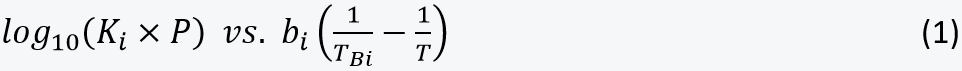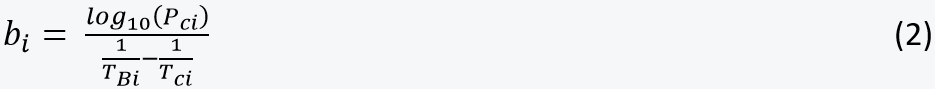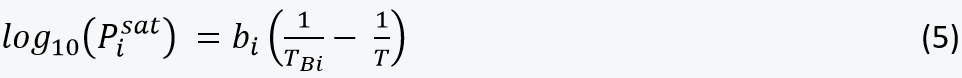•# Tech Talk

## #12 Hoffmann Plots – Theoretical Foundation

PVTsim users often ask why we do not assign any importance to Hoffmann plots in the QC module of PVTsim Nova.

The idea of a Hoffmann plot is to validate whether a gas and a liquid separator sample are in equilibrium at separator conditions.

The below table shows a recombined reservoir fluid composition for a volatile oil and the separator gas and liquid compositions at 69 bar and 65oC.  The recombined reservoir fluid composition will only be representative of the reservoir fluid if the separator gas and separator liquid compositions are in thermodynamic equilibrium at the separator conditions. It would be useful to have a method to clarify if that is the case.

 Component Recombined Reservoir fluidmol % Separator GasMol% Separator LiquidMol% N2 1.775 2.320 0.226 CO2 2.929 3.390 1.620 H2S 11.450 10.669 13.667 C1 61.314 76.443 18.340 C2 2.707 2.873 2.235 C3 2.145 1.786 3.166 iC4 0.772 0.498 1.550 nC4 1.521 0.851 3.426 iC5 0.872 0.332 2.407 nC5 0.872 0.289 2.527 C6 1.470 0.268 4.885 C7 1.666 0.134 5.871 C8 1.783 0.083 6.529 C9 1.520 0.040 5.609 C10+ 7.204 0.025 27.944

Recombined reservoir fluid and separator gas and liquid compositions at 69 bar and 65 oC. The recombined fluid has a C7+ molecular weight of 181.5 and a C7+ density of 0.824 g/cm

The method of Hoffmann et al. was  published in 1953 in a paper entitled “Equilibrium Constants for a Gas-Condensate System” (Petroleum Transactions, AIME).

We have dug into the theory behind Hoffmann plots and found it is based on simple and approximate correlations that are otherwise no longer used in oil industry.

The Hoffmann plot assumes that a linear relation exists forwhere i is a component index andK             Equilibrium constant (ratio of component mole fractions in gas and liquid)
P             Pressure
Pc            Critical pressure
T              Absolute temperature
TB            Boiling temperature at atmospheric pressure
Tc            Critical temperature

A Hoffmann plot for the separator gas and liquid in the above table is shown below. A perfect Hoffmann plot would have all the blue dots on the orange line, which criterion is not completely fulfilled on the below plotHoffmann plot for volatile oil separated at 69 bar and 65oC.

To arrive at the linearity in Equation (1), the pure component vapor pressure of component i is assumed to follow the below simplified Antoine equation (1888)where ai and bi are constants specific for component i.

At the normal boiling point (TB) the vapor pressure equals atmospheric pressure (1 atm), givingThis allows the Antoine equation to be rewritten toAt the critical point the vapor pressure equals Pc and the temperature is Tc. This gives the following expression for biFor an ideal liquid mixture and an ideal gas, the K-factor of component i can be calculated from Raoult’s law (1887)which meansAn ideal mixture is one, where there is no difference between the interaction between two molecules of the same chemical species and two molecules of different chemical species.

Combining Equation (8) and Equation (5) givesThis is a special case of the Hoffmann relation where the straight line assumed in the Hoffmann method follows the equation Y=X. The right-hand side is independent of pressure, and a pressure correction is needed to arrive at the final Hoffmann relation.

Poynting (~1900) introduced a pressure correction through what he called a modified saturation pressure as defined below. It was later known as the pure component fugacityis a so-called fugacity coefficient. Replacingin Equation (8) byassumewhere Con_A and Con_B are constants independent of component index. Combined with Equation (5)  that giveswhich reproduces Equation (1).

Several questionable approximations have been made, which makes the Hoffmann relation highly uncertain:

• The pure component vapor pressure does not in general follow the Antoine equation
• A separator gas is not an ideal gas
• A separator oil is not an ideal mixture.
• The Poynting correction is an approximation and component dependent.

Looking at the above Hoffmann plot, one may get the impression that the assumed linear relation is close to being fulfilled, but that is not quite true. The below table shows the composition of the sampled separator gas and of the gas composition you would get with the Hoffman relation, i.e. if all the blue dots on the above Hoffman plot were moved to be on the orange line. The Hoffmann gas composition deviates significantly from the measured one.

 Component Separator Gas Mol% Hoffmann Gas Mol% %Dev N2 2.32 1.735 -25.2 CO2 3.39 3.015 -11.1 H2S 10.669 10.670 0.0 C1 76.443 78.383 2.5 C2 2.873 2.642 -8.0 C3 1.786 1.497 -16.2 iC4 0.498 0.387 -22.3 nC4 0.851 0.698 -18.0 iC5 0.332 0.256 -22.8 nC5 0.289 0.221 -23.5 C6 0.268 0.212 -20.9

Measured separator gas composition at 69 bar and 65oC and the one that obeys the Hoffmann relation.

The Hoffmann method was good practice at a time when computers and cubic equations of state (EoS) had not yet made their way into oil industry. Cubic equations were known at the time Hoffmann presented his method, but phase equilibrium calculations using cubic equations were out of reach until computers in the seventies became a widely used engineering tool in oil industry.

Today, more refined EoS-based QC techniques exist. Within seconds, it is possible to perform EoS-based flash and phase equilibrium calculations, which are much more accurate than the correlations forming the basis of the Hoffmann plot.

The below plot shows phase envelopes of the separator gas and separator liquid simulated using a cubic EoS. To be in equilibrium at the separator conditions the phase envelopes must have a point of intersection at the separator temperature and pressure (65oC and 69 bar), which is seen to be the case.QC check for separator conditions. The phase envelopes of a gas and liquid in equilibrium at the separator conditions must intersect at the separator T and P.

With a cubic equation, it is straight forward to perform a PT flash calculation of the recombined reservoir fluid composition at separator conditions and compare the simulated K-factors with those derived from the sampled separator composition. A plot of experimental K-factors versus simulated K-factors should give a straight line with  Y=X as illustrated in the below figure.Experimental versus simulated K-factors at separator conditions.

The two latter plots are examples of the QC checks performed by the QC module in PVTsim Nova.